# C Program to Print Diamond Pattern

In this tutorial you will learn about the C Program to Print Diamond Pattern and its application with practical example.

## C Program to Print Diamond Pattern

In this tutorial, we will learn to create a C program that will create a diamond pattern using C programming.

## Prerequisites

Before starting with this tutorial we assume that you are best aware of the following C programming topics:

• Operators in C Programming.
• Basic Input and Output function in C Programming.
• Basic C programming.
• For loop in C Programming.

## Create a diamond:-

The C language is a very powerful programming language. In C programming we can perform many operations with the help of codings. The c language is very easy to create any pattern. In this program, we will learn to create diamond-like patterns with the help of some code.

## Program:-

To create a diamond pattern

## Output:-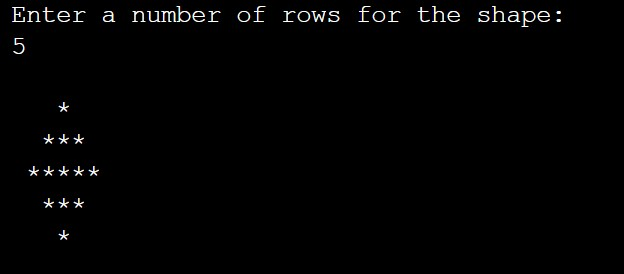The above program we have first initialize the required variable.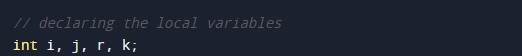• i = it will hold the integer value to control parent for loop.
• j = it will hold the integer value to control child for loop.
• k = it will hold the integer value to control child for loop.
• r = it will hold the integer value for the number of rows of pattern.

Initializing the first parent for loop size and number of rows.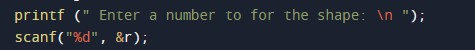For Loop to print the blank spaces.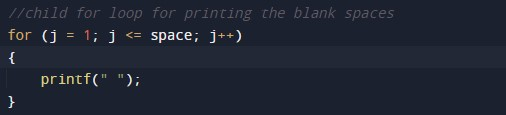For Loop to print the “*”.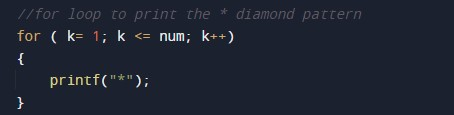Main Program Code.In this tutorial we have learn about the C Program to Print Diamond Pattern and its application with practical example. I hope you will like this tutorial.